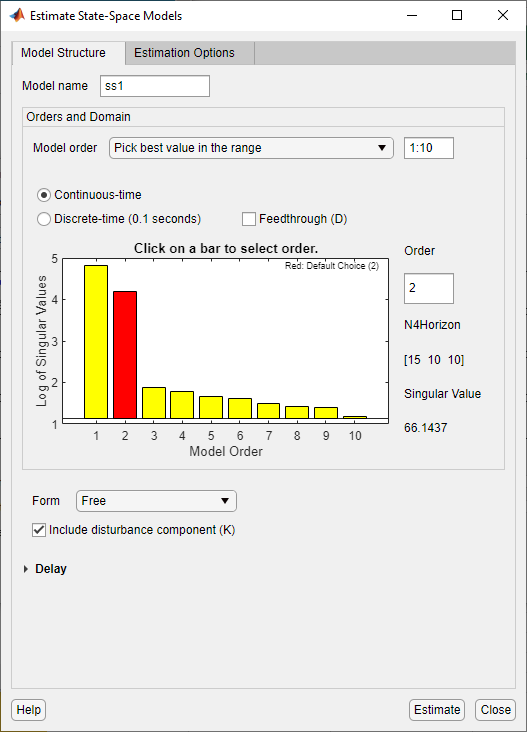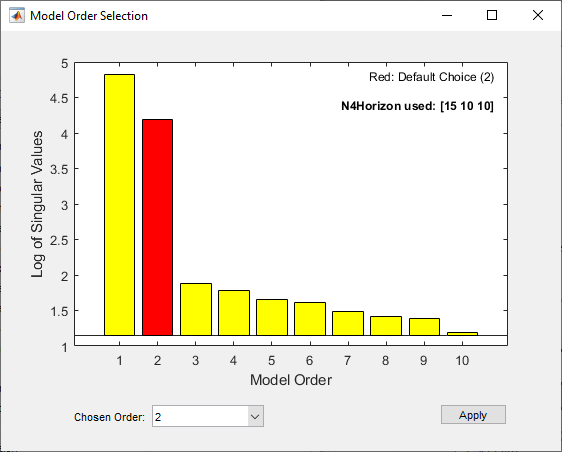## Estimate State-Space Model With Order Selection

To estimate a state-space model, you must provide a value of its order, which represents the number of states. When you do not know the order, you can search and select an order using the following procedures.

### Estimate Model With Selected Order in the App

You must have already imported your data into the app, as described in Represent Data.

To estimate model orders for a specific model structure and configuration:

1. In the System Identification app, select Estimate > State Space Models to open the State Space Models dialog box.

2. In the Model Structure tab, select the Pick best value in the range option and specify a range in the adjacent field. The default range is `1:10`.

This action opens the Model Order Selection window, which displays the relative measure of how much each state contributes to the input-output behavior of the model (log of singular values of the covariance matrix). The following figure shows an example plot. In this figure, states 1 and 2 provide the most significant contribution. The contributions to the right of state 2 drop significantly. The red bar illustrates the cutoff. The order of this bar represents the best-value recommendation, and this value appears in Order. You can override the recommendation by clicking on another bar or by overwriting the contents of Order. For information about using the Model Order Selection window, see Using the Model Order Selection Window3. (Optional) Specify additional attributes of the model structure, such as input delay and feedthrough. You can also modify the estimation options in the Estimation Options tab. As you modify your selections, the software re-evaluates the model-order recommendation.

4. Click Estimate. This action adds a new model to the Model Board in the System Identification app. The default name of the model is `ss1`. You can use this model as an initial guess for estimating other state-space models, as described in Estimate State-Space Models in System Identification App.

5. Click to close the window.

### Estimate Model With Selected Order at the Command Line

You can estimate a state-space model with selected order using `n4sid`, `ssest` or `ssregest`.

Use the following syntax to specify the range of model orders to try for a specific input delay:

`m = n4sid(data,n1:n2);`

where `data` is the estimation data set, `n1` and `n2` specify the range of orders.

The command opens the Model Order Selection window. For information about using this plot, see Using the Model Order Selection Window.

Alternatively, use `ssest` or `ssregest`:

```m1 = ssest(data,nn) m2 = ssregest(data,nn)```

where `nn = [n1,n2,...,nN]` specifies the vector or range of orders you want to try.

`n4sid` and `ssregest` estimate a model whose sample time matches that of `data` by default, hence a discrete-time model for time-domain data. `ssest` estimates a continuous-time model by default. You can change the default setting by including the `Ts` name-value pair input arguments in the estimation command. For example, to estimate a discrete-time model of optimal order, assuming `Data.Ts>0`, type:

`model = ssest(data,nn,'Ts',data.Ts);`

or

`model = ssregest(data,nn,'Ts',data.Ts);`

To automatically select the best order without opening the Model Order Selection window, type `m = n4sid(data,'best')`, `m = ssest(data,'best')` or `m = ssregest(data,'best')`.

### Using the Model Order Selection Window

The following figure shows a sample Model Order Selection window.You use this plot to decide which states provide a significant relative contribution to the input-output behavior, and which states provide the smallest contribution. Based on this plot, select the rectangle that represents the cutoff for the states on the left that provide a significant contribution to the input-output behavior. The recommended choice is shown in red. To learn how to generate this plot, see Estimate Model With Selected Order in the App or Estimate Model With Selected Order at the Command Line.

The horizontal axis corresponds to the model order `n`. The vertical axis, called Log of Singular values, shows the singular values of a covariance matrix constructed from the observed data.

For example, in the previous figure, states 1 and 2 provide the most significant contribution. However, the contributions of the states to the right of state 2 drop significantly. This sharp decrease in the log of the singular values after `n`=2 indicates that using two states is sufficient to get an accurate model.

## Support